# Exterior Angle Theorem

For a triangle: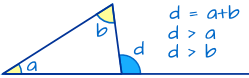• The exterior angle d equals the angles a plus b.
• The exterior angle d is greater than angle a, or angle b.

### Example: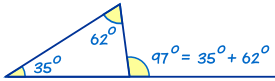The exterior angle is 35° + 62° = 97°

And 97° > 35°

And 97° > 62°

## Why?

Because the interior angles of a triangle add to 180°, and angles c+d also add to 180°: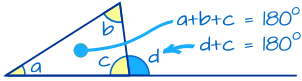The interior angles of a triangle add to 180°:a + b + c = 180°
Angles c and d make a straight angle, which is 180°:d + c = 180°
So d + c equals a + b + c:d + c = a + b + c
Subtract c from both sides:d = a + b

## Works For Any Triangle's Exterior Angle

### Example: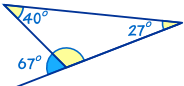The exterior angle is 40° + 27° = 67°

And 67° > 40°

And 67° > 27°

### Example: How big is angle d?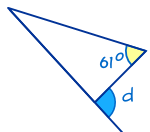We can't calculate exactly, but we can say:

> 61°